Скачать презентацию The Value of Common Stocks Oh the ragman

• Количество слайдов: 22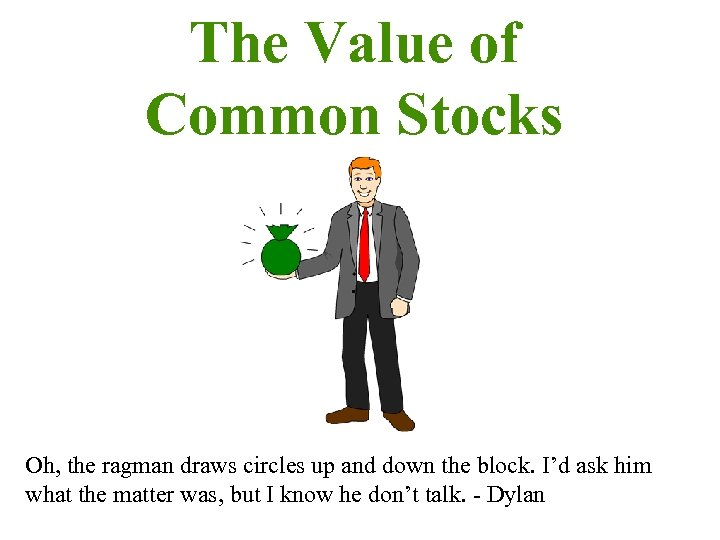The Value of Common Stocks Oh, the ragman draws circles up and down the block. I’d ask him what the matter was, but I know he don’t talk. - Dylan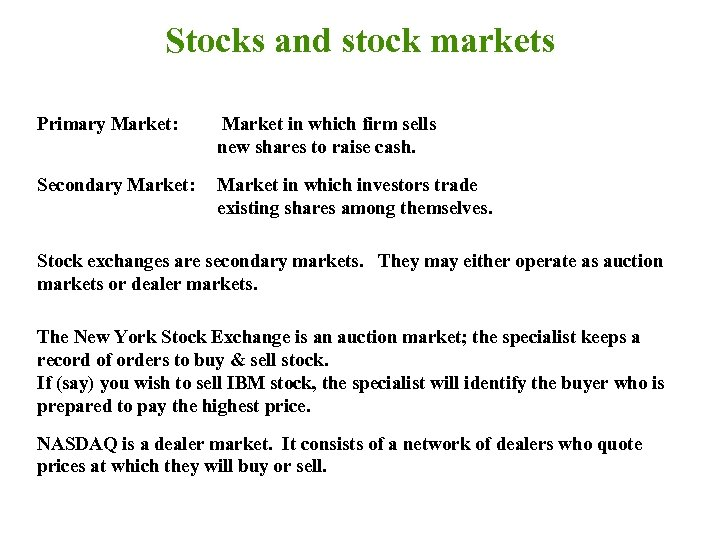Stocks and stock markets Primary Market: Market in which firm sells new shares to raise cash. Secondary Market: Market in which investors trade existing shares among themselves. Stock exchanges are secondary markets. They may either operate as auction markets or dealer markets. The New York Stock Exchange is an auction market; the specialist keeps a record of orders to buy & sell stock. If (say) you wish to sell IBM stock, the specialist will identify the buyer who is prepared to pay the highest price. NASDAQ is a dealer market. It consists of a network of dealers who quote prices at which they will buy or sell.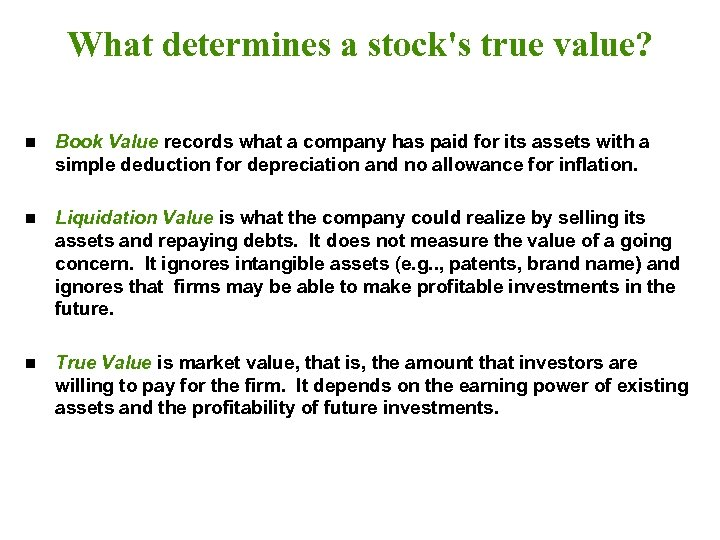What determines a stock's true value? n Book Value records what a company has paid for its assets with a simple deduction for depreciation and no allowance for inflation. n Liquidation Value is what the company could realize by selling its assets and repaying debts. It does not measure the value of a going concern. It ignores intangible assets (e. g. . , patents, brand name) and ignores that firms may be able to make profitable investments in the future. n True Value is market value, that is, the amount that investors are willing to pay for the firm. It depends on the earning power of existing assets and the profitability of future investments.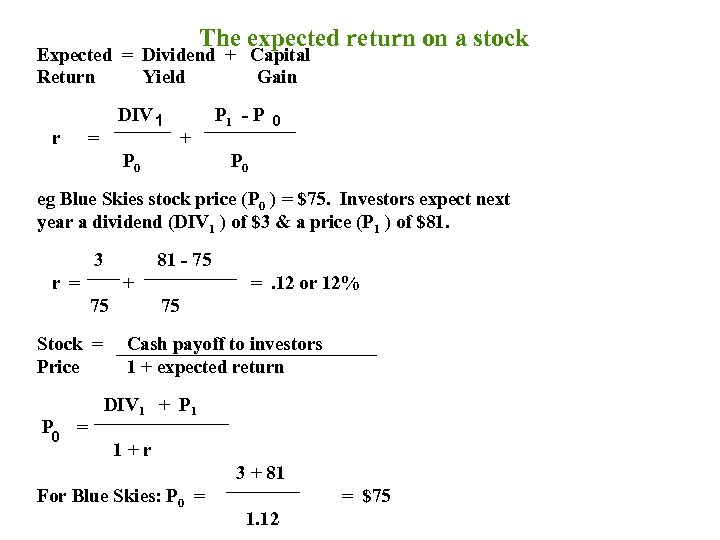The expected return on a stock Expected = Dividend + Capital Return Yield Gain r DIV 1 = + P 0 P 1 - P 0 eg Blue Skies stock price (P 0 ) = \$75. Investors expect next year a dividend (DIV 1 ) of \$3 & a price (P 1 ) of \$81. 3 81 - 75 r = + 75 Stock = Price P 0 = =. 12 or 12% 75 Cash payoff to investors 1 + expected return DIV 1 + P 1 1+r 3 + 81 For Blue Skies: P 0 = = \$75 1. 12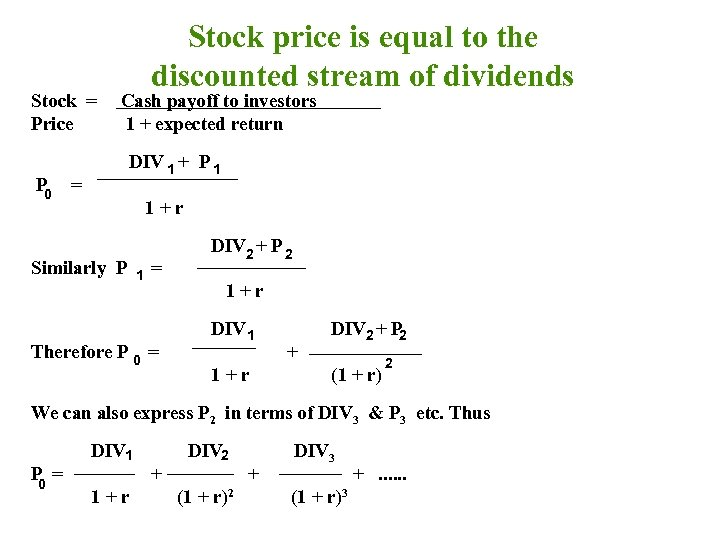Stock = Price P 0 Stock price is equal to the discounted stream of dividends Cash payoff to investors 1 + expected return DIV 1 + P 1 = 1+r Similarly P 1 = DIV 2 + P 2 1+r Therefore P 0 = DIV 1 1+r + DIV 2 + P 2 (1 + r) 2 We can also express P 2 in terms of DIV 3 & P 3 etc. Thus P = 0 DIV 1 1+r + DIV 2 (1 + r)2 + DIV 3 (1 + r)3 +. . .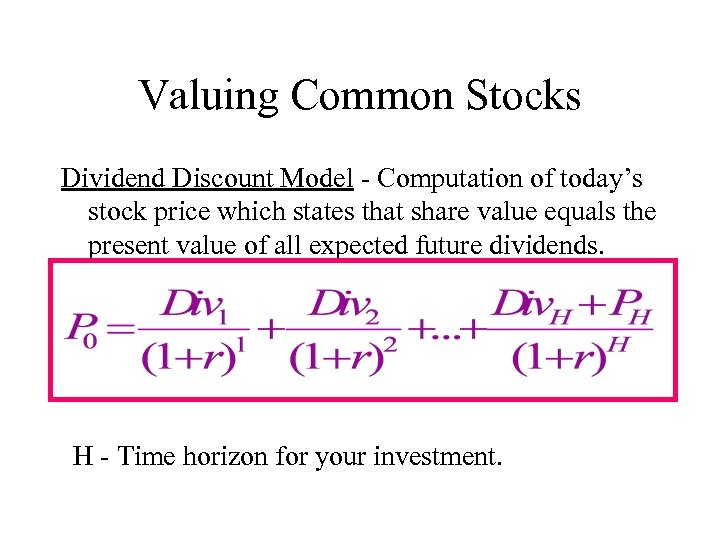Valuing Common Stocks Dividend Discount Model - Computation of today’s stock price which states that share value equals the present value of all expected future dividends. H - Time horizon for your investment.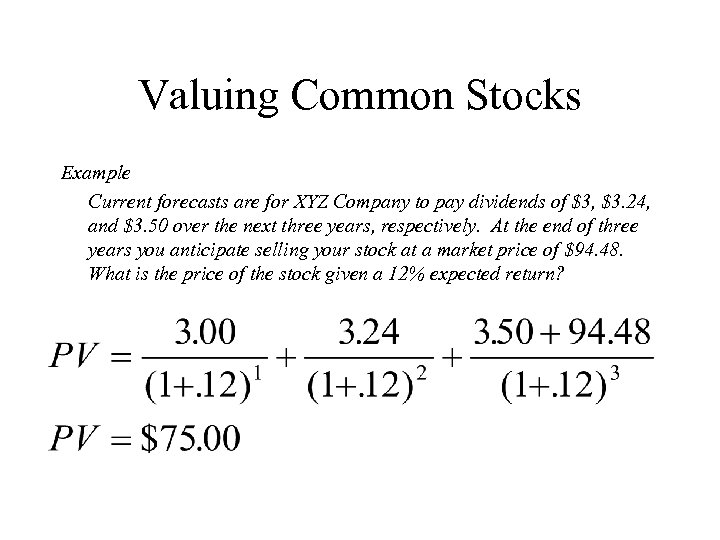Valuing Common Stocks Example Current forecasts are for XYZ Company to pay dividends of \$3, \$3. 24, and \$3. 50 over the next three years, respectively. At the end of three years you anticipate selling your stock at a market price of \$94. 48. What is the price of the stock given a 12% expected return?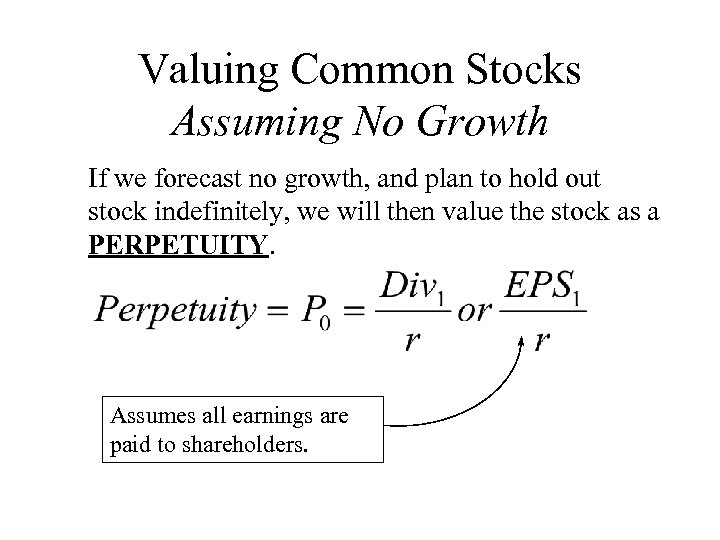Valuing Common Stocks Assuming No Growth If we forecast no growth, and plan to hold out stock indefinitely, we will then value the stock as a PERPETUITY. Assumes all earnings are paid to shareholders.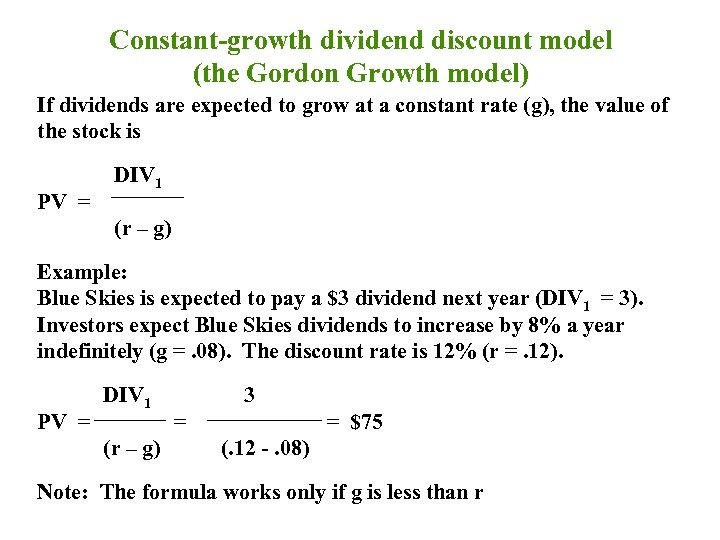Constant-growth dividend discount model (the Gordon Growth model) If dividends are expected to grow at a constant rate (g), the value of the stock is PV = DIV 1 (r – g) Example: Blue Skies is expected to pay a \$3 dividend next year (DIV 1 = 3). Investors expect Blue Skies dividends to increase by 8% a year indefinitely (g =. 08). The discount rate is 12% (r =. 12). PV = DIV 1 (r – g) 3 = = \$75 (. 12 -. 08) Note: The formula works only if g is less than r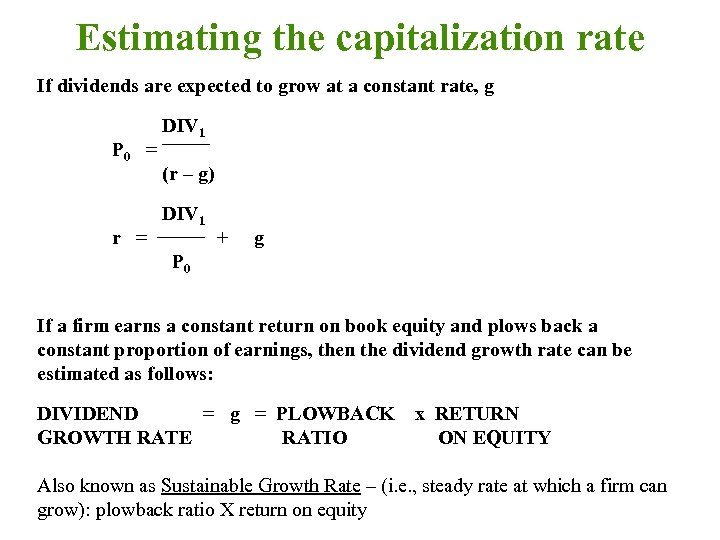Estimating the capitalization rate If dividends are expected to grow at a constant rate, g P 0 = r = DIV 1 (r – g) DIV 1 + g P 0 If a firm earns a constant return on book equity and plows back a constant proportion of earnings, then the dividend growth rate can be estimated as follows: DIVIDEND = g = PLOWBACK GROWTH RATE RATIO x RETURN ON EQUITY Also known as Sustainable Growth Rate – (i. e. , steady rate at which a firm can grow): plowback ratio X return on equity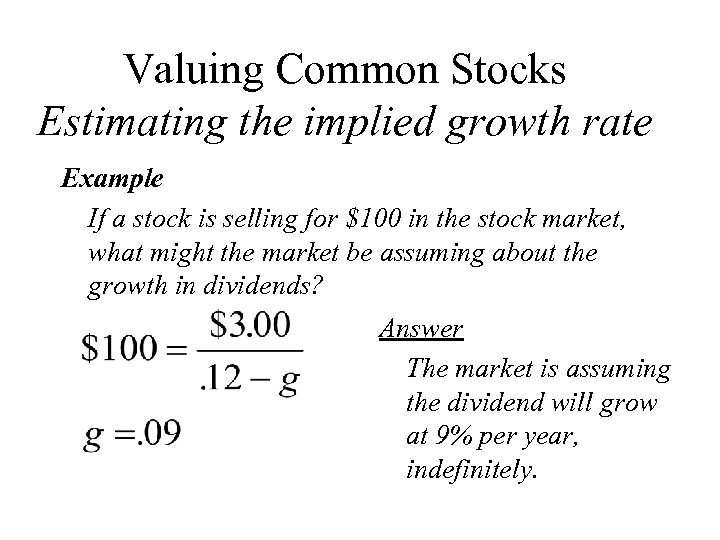Valuing Common Stocks Estimating the implied growth rate Example If a stock is selling for \$100 in the stock market, what might the market be assuming about the growth in dividends? Answer The market is assuming the dividend will grow at 9% per year, indefinitely.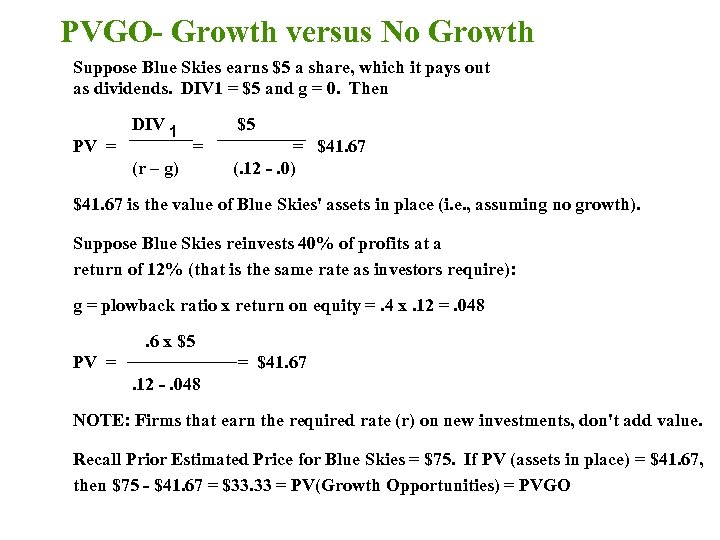PVGO- Growth versus No Growth Suppose Blue Skies earns \$5 a share, which it pays out as dividends. DIV 1 = \$5 and g = 0. Then PV = DIV 1 \$5 = (r – g) = \$41. 67 (. 12 -. 0) \$41. 67 is the value of Blue Skies' assets in place (i. e. , assuming no growth). Suppose Blue Skies reinvests 40% of profits at a return of 12% (that is the same rate as investors require): g = plowback ratio x return on equity =. 4 x. 12 =. 048. 6 x \$5 PV = = \$41. 67. 12 -. 048 NOTE: Firms that earn the required rate (r) on new investments, don't add value. Recall Prior Estimated Price for Blue Skies = \$75. If PV (assets in place) = \$41. 67, then \$75 - \$41. 67 = \$33. 33 = PV(Growth Opportunities) = PVGO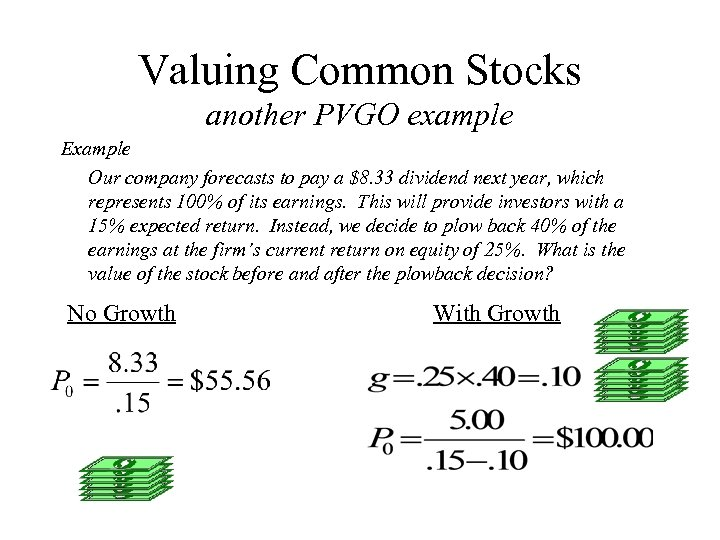Valuing Common Stocks another PVGO example Example Our company forecasts to pay a \$8. 33 dividend next year, which represents 100% of its earnings. This will provide investors with a 15% expected return. Instead, we decide to plow back 40% of the earnings at the firm’s current return on equity of 25%. What is the value of the stock before and after the plowback decision? No Growth With Growth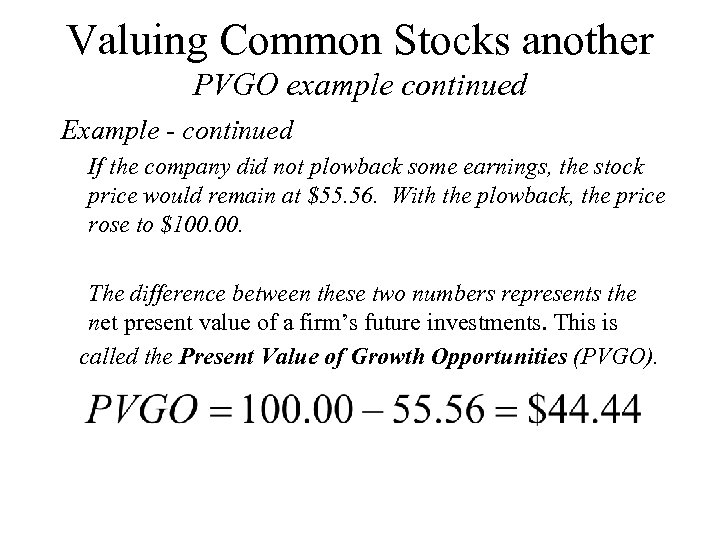Valuing Common Stocks another PVGO example continued Example - continued If the company did not plowback some earnings, the stock price would remain at \$55. 56. With the plowback, the price rose to \$100. The difference between these two numbers represents the net present value of a firm’s future investments. This is called the Present Value of Growth Opportunities (PVGO).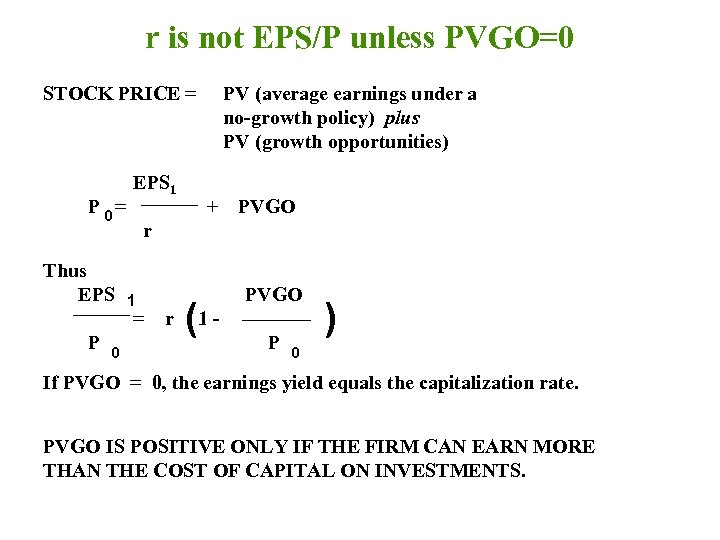r is not EPS/P unless PVGO=0 STOCK PRICE = P 0= Thus EPS 1 0 + PVGO r 1 = P PV (average earnings under a no-growth policy) plus PV (growth opportunities) r (1 - PVGO P ) 0 If PVGO = 0, the earnings yield equals the capitalization rate. PVGO IS POSITIVE ONLY IF THE FIRM CAN EARN MORE THAN THE COST OF CAPITAL ON INVESTMENTS.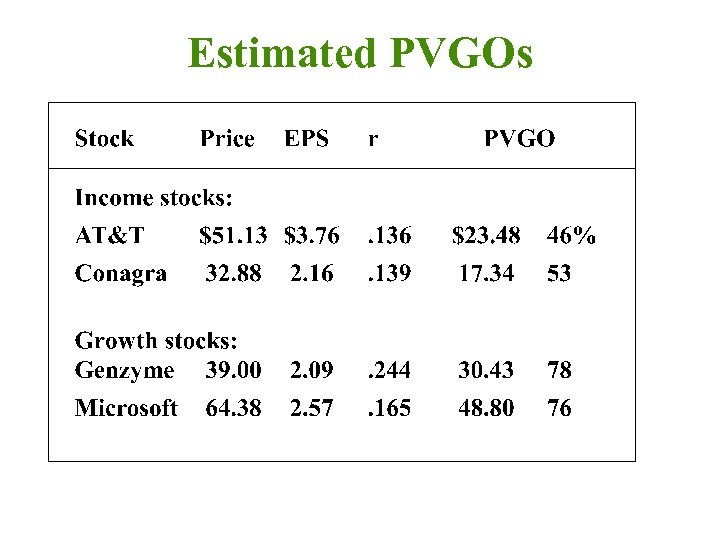Estimated PVGOs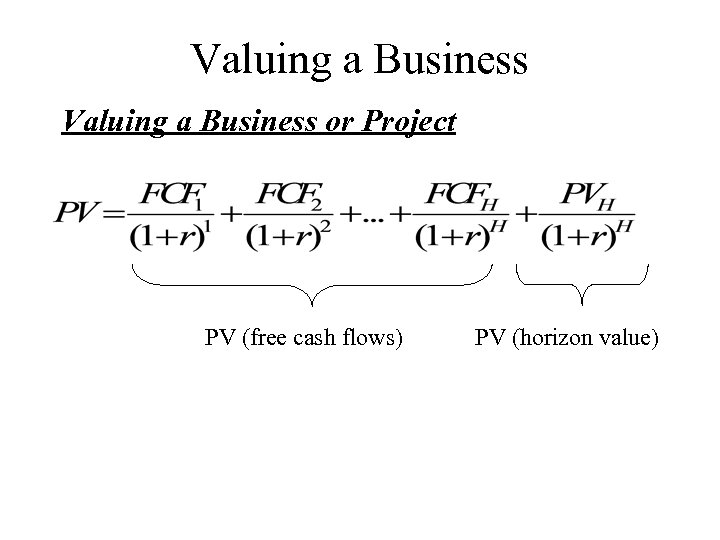Valuing a Business or Project PV (free cash flows) PV (horizon value)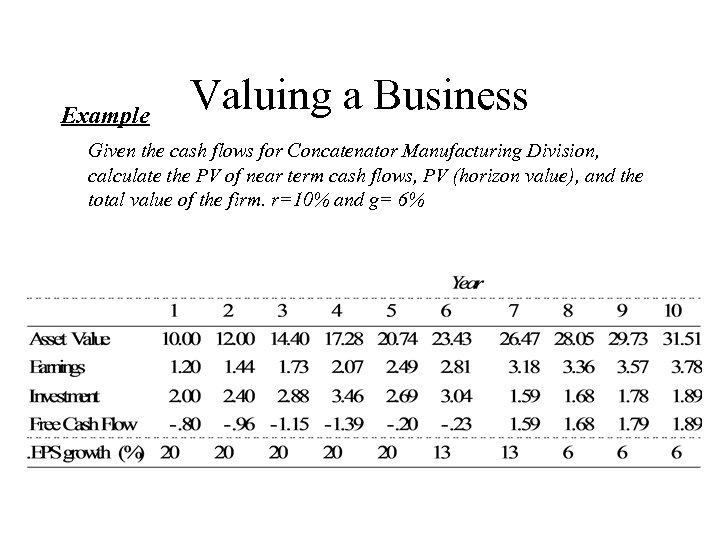Example Valuing a Business Given the cash flows for Concatenator Manufacturing Division, calculate the PV of near term cash flows, PV (horizon value), and the total value of the firm. r=10% and g= 6%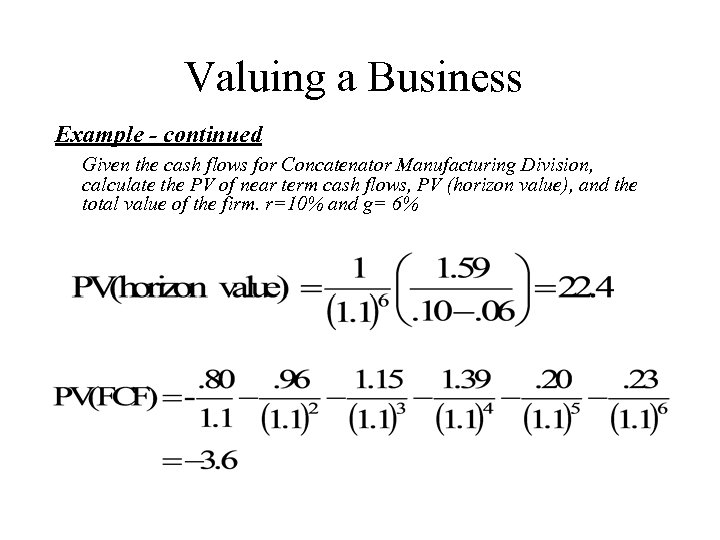Valuing a Business Example - continued Given the cash flows for Concatenator Manufacturing Division, calculate the PV of near term cash flows, PV (horizon value), and the total value of the firm. r=10% and g= 6%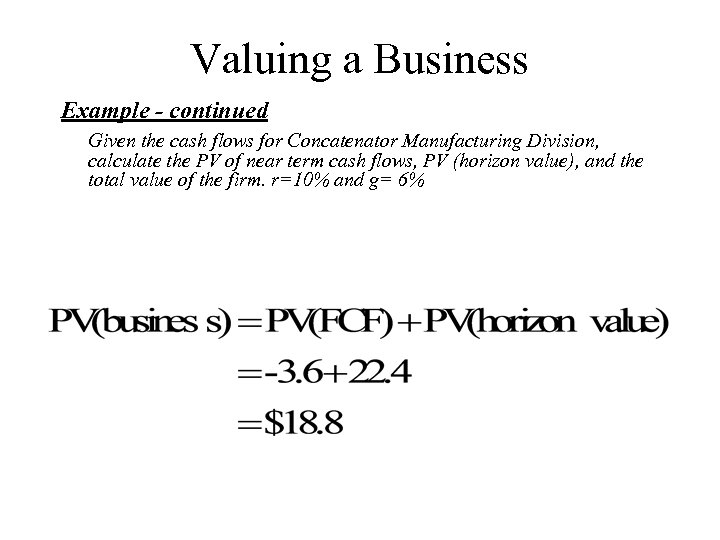Valuing a Business Example - continued Given the cash flows for Concatenator Manufacturing Division, calculate the PV of near term cash flows, PV (horizon value), and the total value of the firm. r=10% and g= 6%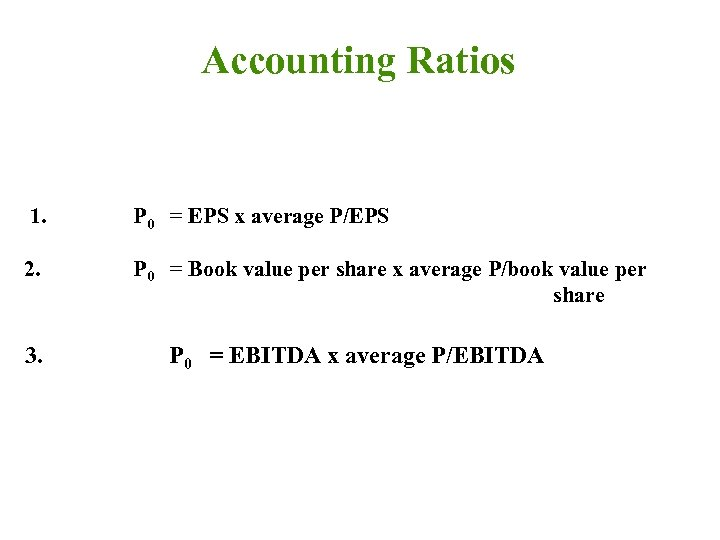Accounting Ratios 1. P 0 = EPS x average P/EPS 2. P 0 = Book value per share x average P/book value per share 3. P 0 = EBITDA x average P/EBITDA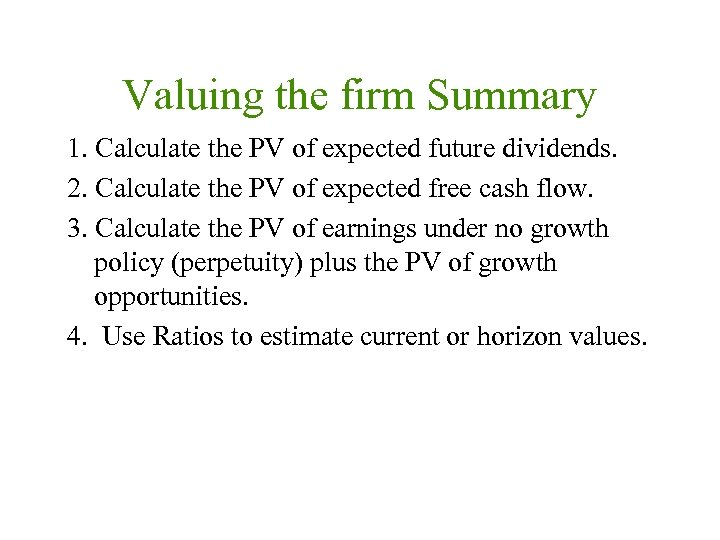Valuing the firm Summary 1. Calculate the PV of expected future dividends. 2. Calculate the PV of expected free cash flow. 3. Calculate the PV of earnings under no growth policy (perpetuity) plus the PV of growth opportunities. 4. Use Ratios to estimate current or horizon values.